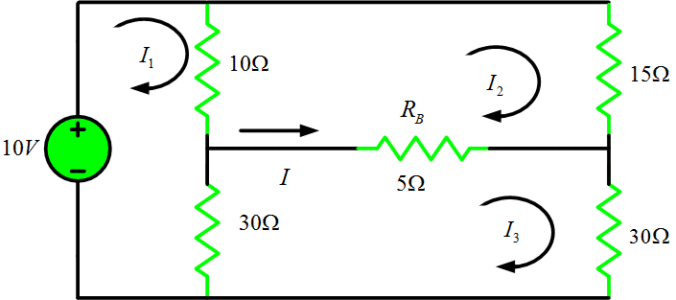Home / Circuits with Matlab / Mesh Analysis using Matlab | Loop Analysis using Matlab

# Mesh Analysis using Matlab | Loop Analysis using Matlab

Want create site? Find Free WordPress Themes and plugins.

In this tutorial, we will find current which is flowing through resistor RB and the power supplied by the voltage source of 10V.First, let’s assign currents for each loop as I1, I2 and I3 and the power supplied by the source is 10*I1 as we can see from the circuit.

Now, let’s write the loop equations for each loop:

Loop 1:

\begin{align} & 10({{I}_{1}}-{{I}_{2}})+30({{I}_{1}}-{{I}_{3}})-10=0 \\ & \begin{matrix} 40{{I}_{1}}-10{{I}_{2}}-30{{I}_{3}}=10 & \cdots & (1) \\\end{matrix} \\\end{align}

Loop 2:

\begin{align} & 10({{I}_{2}}-{{I}_{1}})+15{{I}_{2}}+5({{I}_{2}}-{{I}_{3}})=0 \\ & \begin{matrix} -10{{I}_{1}}+30{{I}_{2}}-5{{I}_{3}}=0 & \cdots & (2) \\\end{matrix} \\\end{align}

Loop 3:

\begin{align} & 30({{I}_{3}}-{{I}_{1}})+5({{I}_{3}}-{{I}_{2}})+30{{I}_{3}}=0 \\ & \begin{matrix} -30{{I}_{1}}-5{{I}_{2}}+65{{I}_{3}}=0 & \cdots & (3) \\\end{matrix} \\\end{align}

Now, let’s write (1), (2), and (3) in matrix form as:

$\left[ \begin{matrix} 40 & -10 & -30 \\ -10 & 30 & -5 \\ -30 & -5 & 65 \\\end{matrix} \right]\left[ \begin{matrix} {{I}_{1}} \\ {{I}_{2}} \\ {{I}_{3}} \\\end{matrix} \right]=\left[ \begin{matrix} 10 \\ 0 \\ 0 \\\end{matrix} \right]$

Now, we will write a small piece of Matlab code to compute all loop currents, a current through RB and power supplied by the voltage source.

clear all;close all;clc
% Loop Analysis using Matlab
R_Mat = [40 -10 -30;
-10 30 -5; % Impedance (or Resistance) Matrix obtain from Loop equations
-30 -5 65];
V_vec = [10 0 0]'; % Voltage Vector (again from Loop equations)
%% % Loop Currents Calculations
I_Loop = inv(R_Mat)*V_vec;
% Calculate current flowing through Resistor R_B
I_RB = I_Loop(3) - I_Loop(2); % (I=I3-I2)
fprintf('The current flowing through Resistor R_B is %8.3f A \n',I_RB)
% Calculate the total power supplied by 10V source
P_Source = I_Loop(1)*10; % (P=10*I_1)
fprintf('The power supplied by voltage source of 10V is %8.4f watts \n',P_Source)


Results:

The current flowing through Resistor R_B is    0.037 A

The power supplied by voltage source of 10V is   4.7531 watts

Did you find apk for android? You can find new Free Android Games and apps.